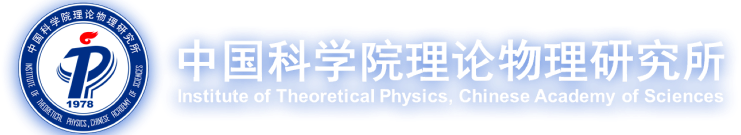#### 学术活动

06/21 2022 Seminar
• Title题目 The analogy of LV fermion-gravity to fermion-photon couplings
• Speaker报告人 肖智 (华北电力大学)
• Date日期 2022年6月21日 15:00
• Venue地点 南楼6620
• Abstract摘要
By adopting a methodology proposed by R.J. Adler et al, we study the interesting analogy between the fermion-gravity and the fermion-electromagnetic interactions in the presence of the minimal Lorentz-violating (LV) fermion coefficients. The one-fermion matrix elements of gravitational interaction (OMEGI) are obtained with a prescribed Lense-Thirring (LT) metric assuming test particle assumption. Quite distinct from the extensively studied linear gravitational potential, the LT metric is an essentially curved metric, and thus reveals the anomalous LV matter-gravity couplings as a manifestation of the so-called gravito-magnetic effects, which go beyond the conventional equivalence principle predictions. By collecting all the spin-dependent operators from the OMEGI with some reasonable assumptions, we get a LV non-relativistic Hamiltonian, from which we derive the anomalous spin precession and gravitational acceleration due to LV. Combined these results with certain spin gravity experiments, we get some rough bounds on several LV parameters, such as the constraint on a combination of the b and H coefficients to the level of 10meV, with some ad hoc assumptions.